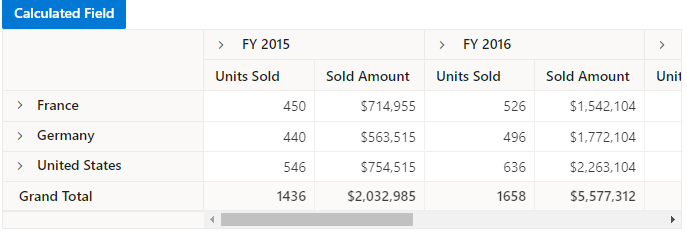# Getting Started with ASP.NET MVC Pivot Table Control

30 May 202224 minutes to read

This section briefly explains about how to include ASP.NET MVC Pivot Table control in your ASP.NET MVC application using Visual Studio.

## Prerequisites

System requirements for ASP.NET MVC controls

## Install ASP.NET MVC package in the application

Syncfusion ASP.NET MVC controls are available in nuget.org. Refer to NuGet packages topic to learn more about installing NuGet packages in various OS environments. To add ASP.NET MVC controls in the application, open the NuGet package manager in Visual Studio (Tools → NuGet Package Manager → Manage NuGet Packages for Solution), search for Syncfusion.EJ2.MVC5 and then install it.

The Syncfusion.EJ2.MVC5 NuGet package has dependencies, Newtonsoft.Json for JSON serialization and Syncfusion.Licensing for validating Syncfusion license key.

If you create ASP.NET MVC application with MVC4 package, search for Syncfusion.EJ2.MVC4 and then install it.

Add Syncfusion.EJ2 namespace reference in `Web.config` under `Views` folder.

``````<namespaces>
</namespaces>
``````

Checkout the Themes topic to learn different ways (CDN, NPM package, and CRG) to refer styles in ASP.NET MVC application, and to have the expected appearance for Syncfusion ASP.NET MVC controls. Here, the theme is referred using CDN inside the `<head>` of `~/Views/Shared/_Layout.cshtml` file as follows,

``````<head>
...
<!-- Syncfusion ASP.NET MVC controls styles -->

In this getting started walk-through, the required scripts are referred using CDN inside the `<head>` of `~/Views/Shared/_Layout.cshtml` file as follows,

``````<head>
...
<!-- Syncfusion ASP.NET MVC controls scripts -->
<script src="https://cdn.syncfusion.com/ej2/20.1.55/dist/ej2.min.js"></script>

## Register Syncfusion Script Manager

Open `~/Views/Shared/_Layout.cshtml` page and register the script manager `EJS().ScriptManager()` at the end of `<body>` in the ASP.NET MVC application as follows.

``````<body>
...
<!-- Syncfusion ASP.NET MVC Script Manager -->
@Html.EJS().ScriptManager()
</body>``````

## Add ASP.NET MVC Pivot Table control

• Now, add the Syncfusion ASP.NET MVC Pivot Table control in `~/Views/Home/Index.cshtml` page.

• The Pivot Table control further needs to be populated with an appropriate data source. For illustration purpose, a collection of objects mentioning the sales details of certain products over a period and region has been prepared. This sample data is assigned to the pivot table control through DataSource property under PivotViewDataSourceSettings class.

``````@model List<PivotTableSample.Controllers.PivotData>

@Html.EJS().PivotView("PivotView").Height("300").DataSourceSettings(dataSource => dataSource.DataSource((IEnumerable<object>)Model)).Render()``````
``````public ActionResult Index()
{
List<PivotData> pivotData = new List<PivotData>();
pivotData.Add(new PivotData { Sold = 31, Amount = 52824, Country = "France", Products = "Mountain Bikes", Year = "FY 2015", Quarter = "Q1" });
pivotData.Add(new PivotData { Sold = 51, Amount = 86904, Country = "France", Products = "Mountain Bikes", Year = "FY 2015", Quarter = "Q2" });
pivotData.Add(new PivotData { Sold = 90, Amount = 153360, Country = "France", Products = "Mountain Bikes", Year = "FY 2015", Quarter = "Q3" });
pivotData.Add(new PivotData { Sold = 25, Amount = 42600, Country = "France", Products = "Mountain Bikes", Year = "FY 2015", Quarter = "Q4" });
pivotData.Add(new PivotData { Sold = 27, Amount = 46008, Country = "France", Products = "Mountain Bikes", Year = "FY 2016", Quarter = "Q1" });
pivotData.Add(new PivotData { Sold = 49, Amount = 83496, Country = "France", Products = "Mountain Bikes", Year = "FY 2016", Quarter = "Q2" });
pivotData.Add(new PivotData { Sold = 95, Amount = 161880, Country = "France", Products = "Mountain Bikes", Year = "FY 2016", Quarter = "Q3" });
pivotData.Add(new PivotData { Sold = 67, Amount = 114168, Country = "France", Products = "Mountain Bikes", Year = "FY 2016", Quarter = "Q4" });
pivotData.Add(new PivotData { Sold = 75, Amount = 127800, Country = "France", Products = "Mountain Bikes", Year = "FY 2017", Quarter = "Q1" });
pivotData.Add(new PivotData { Sold = 67, Amount = 114168, Country = "France", Products = "Mountain Bikes", Year = "FY 2017", Quarter = "Q2" });
pivotData.Add(new PivotData { Sold = 69, Amount = 117576, Country = "France", Products = "Mountain Bikes", Year = "FY 2017", Quarter = "Q3" });
pivotData.Add(new PivotData { Sold = 90, Amount = 153360, Country = "France", Products = "Mountain Bikes", Year = "FY 2017", Quarter = "Q4" });
pivotData.Add(new PivotData { Sold = 16, Amount = 27264, Country = "France", Products = "Mountain Bikes", Year = "FY 2018", Quarter = "Q1" });
pivotData.Add(new PivotData { Sold = 83, Amount = 124422, Country = "France", Products = "Road Bikes", Year = "FY 2015", Quarter = "Q1" });
pivotData.Add(new PivotData { Sold = 57, Amount = 85448, Country = "France", Products = "Road Bikes", Year = "FY 2015", Quarter = "Q2" });
pivotData.Add(new PivotData { Sold = 20, Amount = 29985, Country = "France", Products = "Road Bikes", Year = "FY 2015", Quarter = "Q3" });
pivotData.Add(new PivotData { Sold = 67, Amount = 70008, Country = "France", Products = "Road Bikes", Year = "FY 2016", Quarter = "Q1" });
pivotData.Add(new PivotData { Sold = 89, Amount = 60496, Country = "France", Products = "Road Bikes", Year = "FY 2016", Quarter = "Q2" });
pivotData.Add(new PivotData { Sold = 75, Amount = 801880, Country = "France", Products = "Road Bikes", Year = "FY 2016", Quarter = "Q3" });
pivotData.Add(new PivotData { Sold = 57, Amount = 204168, Country = "France", Products = "Road Bikes", Year = "FY 2016", Quarter = "Q4" });
pivotData.Add(new PivotData { Sold = 75, Amount = 737800, Country = "France", Products = "Road Bikes", Year = "FY 2017", Quarter = "Q1" });
pivotData.Add(new PivotData { Sold = 87, Amount = 884168, Country = "France", Products = "Road Bikes", Year = "FY 2017", Quarter = "Q2" });
pivotData.Add(new PivotData { Sold = 39, Amount = 729576, Country = "France", Products = "Road Bikes", Year = "FY 2017", Quarter = "Q3" });
pivotData.Add(new PivotData { Sold = 90, Amount = 38860, Country = "France", Products = "Road Bikes", Year = "FY 2017", Quarter = "Q4" });
pivotData.Add(new PivotData { Sold = 93, Amount = 139412, Country = "France", Products = "Road Bikes", Year = "FY 2015", Quarter = "Q4" });
pivotData.Add(new PivotData { Sold = 51, Amount = 92824, Country = "Germany", Products = "Mountain Bikes", Year = "FY 2015", Quarter = "Q1" });
pivotData.Add(new PivotData { Sold = 61, Amount = 76904, Country = "Germany", Products = "Mountain Bikes", Year = "FY 2015", Quarter = "Q2" });
pivotData.Add(new PivotData { Sold = 70, Amount = 43360, Country = "Germany", Products = "Mountain Bikes", Year = "FY 2015", Quarter = "Q3" });
pivotData.Add(new PivotData { Sold = 85, Amount = 62600, Country = "Germany", Products = "Mountain Bikes", Year = "FY 2015", Quarter = "Q4" });
pivotData.Add(new PivotData { Sold = 97, Amount = 86008, Country = "Germany", Products = "Mountain Bikes", Year = "FY 2016", Quarter = "Q1" });
pivotData.Add(new PivotData { Sold = 69, Amount = 93496, Country = "Germany", Products = "Mountain Bikes", Year = "FY 2016", Quarter = "Q2" });
pivotData.Add(new PivotData { Sold = 45, Amount = 301880, Country = "Germany", Products = "Mountain Bikes", Year = "FY 2016", Quarter = "Q3" });
pivotData.Add(new PivotData { Sold = 77, Amount = 404168, Country = "Germany", Products = "Mountain Bikes", Year = "FY 2016", Quarter = "Q4" });
pivotData.Add(new PivotData { Sold = 15, Amount = 137800, Country = "Germany", Products = "Mountain Bikes", Year = "FY 2017", Quarter = "Q1" });
pivotData.Add(new PivotData { Sold = 37, Amount = 184168, Country = "Germany", Products = "Mountain Bikes", Year = "FY 2017", Quarter = "Q2" });
pivotData.Add(new PivotData { Sold = 49, Amount = 89576, Country = "Germany", Products = "Mountain Bikes", Year = "FY 2017", Quarter = "Q3" });
pivotData.Add(new PivotData { Sold = 40, Amount = 33360, Country = "Germany", Products = "Mountain Bikes", Year = "FY 2017", Quarter = "Q4" });
pivotData.Add(new PivotData { Sold = 96, Amount = 77264, Country = "Germany", Products = "Mountain Bikes", Year = "FY 2018", Quarter = "Q1" });
pivotData.Add(new PivotData { Sold = 23, Amount = 24422, Country = "Germany", Products = "Road Bikes", Year = "FY 2015", Quarter = "Q1" });
pivotData.Add(new PivotData { Sold = 67, Amount = 75448, Country = "Germany", Products = "Road Bikes", Year = "FY 2015", Quarter = "Q2" });
pivotData.Add(new PivotData { Sold = 70, Amount = 52345, Country = "Germany", Products = "Road Bikes", Year = "FY 2015", Quarter = "Q3" });
pivotData.Add(new PivotData { Sold = 13, Amount = 135612, Country = "Germany", Products = "Road Bikes", Year = "FY 2015", Quarter = "Q4" });
pivotData.Add(new PivotData { Sold = 57, Amount = 90008, Country = "Germany", Products = "Road Bikes", Year = "FY 2016", Quarter = "Q1" });
pivotData.Add(new PivotData { Sold = 29, Amount = 90496, Country = "Germany", Products = "Road Bikes", Year = "FY 2016", Quarter = "Q2" });
pivotData.Add(new PivotData { Sold = 45, Amount = 301880, Country = "Germany", Products = "Road Bikes", Year = "FY 2016", Quarter = "Q3" });
pivotData.Add(new PivotData { Sold = 77, Amount = 404168, Country = "Germany", Products = "Road Bikes", Year = "FY 2016", Quarter = "Q4" });
pivotData.Add(new PivotData { Sold = 15, Amount = 137800, Country = "Germany", Products = "Road Bikes", Year = "FY 2017", Quarter = "Q1" });
pivotData.Add(new PivotData { Sold = 37, Amount = 184168, Country = "Germany", Products = "Road Bikes", Year = "FY 2017", Quarter = "Q2" });
pivotData.Add(new PivotData { Sold = 99, Amount = 829576, Country = "Germany", Products = "Road Bikes", Year = "FY 2017", Quarter = "Q3" });
pivotData.Add(new PivotData { Sold = 80, Amount = 38360, Country = "Germany", Products = "Road Bikes", Year = "FY 2017", Quarter = "Q4" });
pivotData.Add(new PivotData { Sold = 91, Amount = 67824, Country = "United States", Products = "Mountain Bikes", Year = "FY 2015", Quarter = "Q1" });
pivotData.Add(new PivotData { Sold = 81, Amount = 99904, Country = "United States", Products = "Mountain Bikes", Year = "FY 2015", Quarter = "Q2" });
pivotData.Add(new PivotData { Sold = 70, Amount = 49360, Country = "United States", Products = "Mountain Bikes", Year = "FY 2015", Quarter = "Q3" });
pivotData.Add(new PivotData { Sold = 65, Amount = 69600, Country = "United States", Products = "Mountain Bikes", Year = "FY 2015", Quarter = "Q4" });
pivotData.Add(new PivotData { Sold = 57, Amount = 90008, Country = "United States", Products = "Mountain Bikes", Year = "FY 2016", Quarter = "Q1" });
pivotData.Add(new PivotData { Sold = 29, Amount = 90496, Country = "United States", Products = "Mountain Bikes", Year = "FY 2016", Quarter = "Q2" });
pivotData.Add(new PivotData { Sold = 85, Amount = 391880, Country = "United States", Products = "Mountain Bikes", Year = "FY 2016", Quarter = "Q3" });
pivotData.Add(new PivotData { Sold = 97, Amount = 904168, Country = "United States", Products = "Mountain Bikes", Year = "FY 2016", Quarter = "Q4" });
pivotData.Add(new PivotData { Sold = 85, Amount = 237800, Country = "United States", Products = "Mountain Bikes", Year = "FY 2017", Quarter = "Q1" });
pivotData.Add(new PivotData { Sold = 77, Amount = 384168, Country = "United States", Products = "Mountain Bikes", Year = "FY 2017", Quarter = "Q2" });
pivotData.Add(new PivotData { Sold = 99, Amount = 829576, Country = "United States", Products = "Mountain Bikes", Year = "FY 2017", Quarter = "Q3" });
pivotData.Add(new PivotData { Sold = 80, Amount = 38360, Country = "United States", Products = "Mountain Bikes", Year = "FY 2017", Quarter = "Q4" });
pivotData.Add(new PivotData { Sold = 76, Amount = 97264, Country = "United States", Products = "Mountain Bikes", Year = "FY 2018", Quarter = "Q1" });
pivotData.Add(new PivotData { Sold = 53, Amount = 94422, Country = "United States", Products = "Road Bikes", Year = "FY 2015", Quarter = "Q1" });
pivotData.Add(new PivotData { Sold = 90, Amount = 45448, Country = "United States", Products = "Road Bikes", Year = "FY 2015", Quarter = "Q2" });
pivotData.Add(new PivotData { Sold = 29, Amount = 92345, Country = "United States", Products = "Road Bikes", Year = "FY 2015", Quarter = "Q3" });
pivotData.Add(new PivotData { Sold = 67, Amount = 235612, Country = "United States", Products = "Road Bikes", Year = "FY 2015", Quarter = "Q4" });
pivotData.Add(new PivotData { Sold = 97, Amount = 90008, Country = "United States", Products = "Road Bikes", Year = "FY 2016", Quarter = "Q1" });
pivotData.Add(new PivotData { Sold = 79, Amount = 90496, Country = "United States", Products = "Road Bikes", Year = "FY 2016", Quarter = "Q2" });
pivotData.Add(new PivotData { Sold = 95, Amount = 501880, Country = "United States", Products = "Road Bikes", Year = "FY 2016", Quarter = "Q3" });
pivotData.Add(new PivotData { Sold = 97, Amount = 104168, Country = "United States", Products = "Road Bikes", Year = "FY 2016", Quarter = "Q4" });
pivotData.Add(new PivotData { Sold = 95, Amount = 837800, Country = "United States", Products = "Road Bikes", Year = "FY 2017", Quarter = "Q1" });
pivotData.Add(new PivotData { Sold = 87, Amount = 684168, Country = "United States", Products = "Road Bikes", Year = "FY 2017", Quarter = "Q2" });
pivotData.Add(new PivotData { Sold = 109, Amount = 29576, Country = "United States", Products = "Road Bikes", Year = "FY 2017", Quarter = "Q3" });
return View(pivotData);
}``````

Press Ctrl+F5 (Windows) or +F5 (macOS) to run the app. Then, the Syncfusion ASP.NET MVC Pivot Table control will be rendered in the default web browser.

## Adding fields to row, column, value and filter axes

Now that pivot table is initialized and assigned with sample data, will further move to showcase the component by organizing appropriate fields in row, column, value and filter axes.

In PivotViewDataSourceSettings class, four major axes - Rows, Columns, Values and Filters plays a vital role in defining and organizing fields from the bound data source, to render the entire pivot table component in a desired format.

Rows – Collection of fields that needs to be displayed in row axis of the pivot table.

Columns – Collection of fields that needs to be displayed in column axis of the pivot table.

Values – Collection of fields that needs to be displayed as aggregated numeric values in the pivot table.

Filters - Collection of fields that would act as master filter over the data bound in row, column and value axes of the pivot table.

In-order to define each field in the respective axis, the following basic properties should be set.

• Name: It allows to set the field name from the bound data source. It’s casing should match exactly like in the data source and if not set properly, the pivot table will not be rendered.
• Caption: It allows to set the field caption, which is the alias name of the field that needs to be displayed in the pivot table.
• Type: It allows to set the summary type of the field. By default, SummaryTypes.Sum is applied.

In this illustration, “Year” and “Quarter” are added in column, “Country” and “Products” in row, and “Sold” and “Amount” in value section respectively.

``````@model List<PivotTableSample.Controllers.PivotData>

@Html.EJS().PivotView("PivotView").Height("300").DataSourceSettings(dataSource => dataSource.DataSource((IEnumerable<object>)Model).ExpandAll(false).EnableSorting(true)
.Rows(rows =>
{
}).Columns(columns =>
{
}).Values(values =>
{
})).Render()``````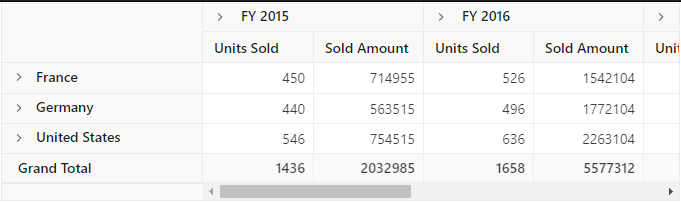## Applying formatting to a value field

Formatting defines a way in which values should be displayed. For example, format “C” denotes the values should be displayed in currency pattern. To do so, define the FormatSetting class with its Name and Format properties and add it to PivotViewFormatSettings. In this illustration, the Name property is set as Amount, a field from value section and its format is set as currency. Likewise, we can set format for other value fields as well and add it to PivotViewFormatSettings.

Only fields from value section, which is in the form of numeric data values are applicable for formatting.

``````@model List<PivotTableSample.Controllers.PivotData>

@Html.EJS().PivotView("PivotView").Height("300").DataSourceSettings(dataSource => dataSource.DataSource((IEnumerable<object>)Model).ExpandAll(false).EnableSorting(true)
.FormatSettings(formatsettings =>
{
}).Rows(rows =>
{
}).Columns(columns =>
{
}).Values(values =>
{
})).Render()``````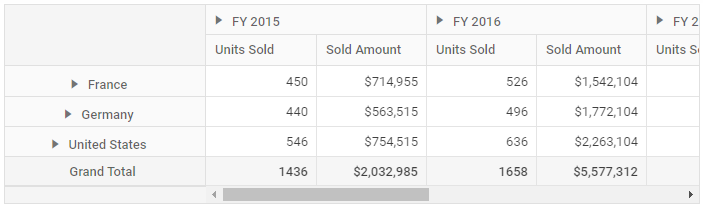## Enable Grouping Bar

The grouping bar feature automatically populates fields from the bound data source and allows end users to drag fields between different axes such as columns, rows, values, and filters, and alter pivot table at runtime. It also provides option to sort, filter and remove fields. It can be enabled by setting the ShowGroupingBar property to true.

``````@model List<PivotTableSample.Controllers.PivotData>

@Html.EJS().PivotView("PivotView").Width("100%").Height("300").DataSourceSettings(dataSource => dataSource.DataSource((IEnumerable<object>)Model).ExpandAll(false).EnableSorting(true).AllowLabelFilter(true).AllowValueFilter(true)
.FormatSettings(formatsettings =>
{
}).Rows(rows =>
{
}).Columns(columns =>
{
}).Values(values =>
{
})).ShowGroupingBar(true).Render()``````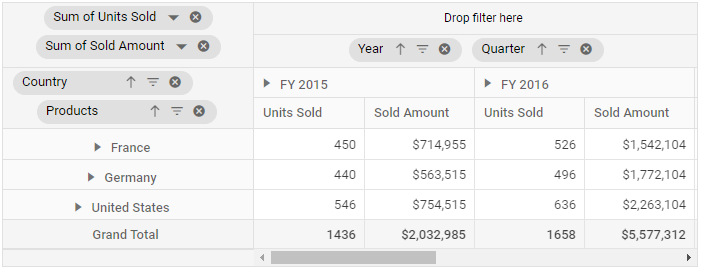## Enable Pivot Field List

The field list allows to add or remove fields and also rearrange the fields between different axes, including column, row, value, and filter along with filter and sort options dynamically at runtime. It can be enabled by setting the ShowFieldList property to true.

``````@model List<PivotTableSample.Controllers.PivotData>

@Html.EJS().PivotView("PivotView").Height("300").DataSourceSettings(dataSource => dataSource.DataSource((IEnumerable<object>)Model).ExpandAll(false).EnableSorting(true).AllowLabelFilter(true).AllowValueFilter(true)
.FormatSettings(formatsettings =>
{
}).Rows(rows =>
{
}).Columns(columns =>
{
}).Values(values =>
{
})).ShowFieldList(true).Render()``````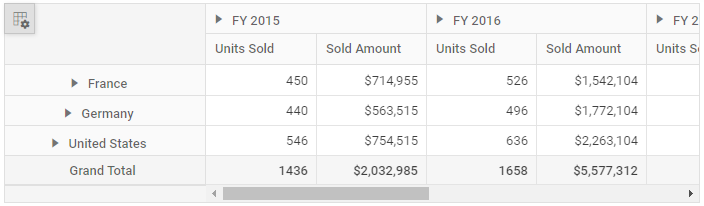## Calculated field

The calculated field feature allows user to insert or add a new calculated field based on the available fields from the bound data source using basic arithmetic operators. The calculated field can be included in pivot table using the CalculatedFieldSetting class from code behind. Or else, calculated fields can be added at run time through the built-in dialog by just setting the AllowCalculatedField property to true in pivot table. You will see a button enabled in the Field List UI automatically to invoke the calculated field dialog and perform necessary operation.

Calculated field is applicable only for value fields.

``````@model List<PivotTableSample.Controllers.PivotData>

@{var amount = "\"" + "Sum(Amount)" + "\"";}
@{var sold = "\"" + "Sum(Sold)" + "\"";}
@{ var totalPrice = amount + "+" + sold;}

@Html.EJS().Button("calculated-field-btn").Content("Calculated Field").IsPrimary(true).Render()
@Html.EJS().PivotView("PivotView").Height("300").DataSourceSettings(dataSource => dataSource.DataSource((IEnumerable<object>)Model).ExpandAll(false).EnableSorting(true)
.FormatSettings(formatsettings =>
{
}).Rows(rows =>
{
}).Columns(columns =>
{
}).Values(values =>
{